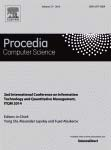• A
• A
• A
• ABC
• ABC
• ABC
• –ź
• –ź
• –ź
• –ź
• –ź
Regular version of the site

## On the Stability of Comparing Histograms with Help of Probabilistic Methods

P. 597-605.

We study the problem of the stability of probabilistic methods of comparing histograms in case of their distortions. The comparing of histograms is given by preorder relation on the set of histograms. This relation should to be agreed with the condition of ordering of arguments histograms by ascendingly their importance. The pointwise interval uncorrelated changes are meant by the distortions of histograms. Necessary and sufficient conditions on the level of distortion of histograms are found under which the comparison of the two histograms is not changed. The study was conducted for three popular probabilistic methods of comparison: a) using the mathematical expectation; b) using the distribution function (stochastic dominance); c) using probability of inequalities. Proved approval illustrated by studies of stability of comparing histograms of USE applicants admitted to universities.

### In bookEdited by: Y. Shi, A. Lepskiy, F. T. Aleskerov. Vol. 31. Amsterdam: Elsevier, 2014.